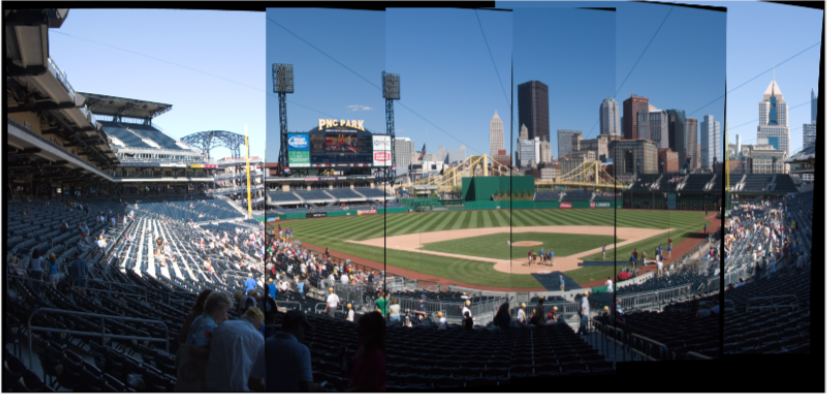CMU

## HomographyThis is an assignment from CMU 16720-A. In this assignment, we are going to implement the algorithm to stitch panoramas. We first understand the theory behinds homographies. Then, we implement our own BRIEF descriptor so that we use these descriptors to do the feature matching. After that, we use those correspondences to get the homography H by using SVD. Finally, we use this H to stitch two images and get the panoramas.

## Theory

In this section, I will not provide detail proof. We first prove that if there is a plane in 3D space and two cameras C1 and C2, then we can find a homography H such that where and . To prove this, we need to use the “planar” property, which means that we need to assume the z value of the 3D homogeneous coordinates to be zero. Then we can reduce the camera matrix from 3×4 to 3×3 so that it is invertible. Next, we will answer the DoF of this H (8) and the point pairs are required to solve h (4).

## BRIEF descriptor

Now, we are going to implement the BRIEF descriptor. We first randomly select 256 (given) position pairs in a 9×9 patch (given). For example, since the patch size is 9×9, we will have 81 pixels, say [1,2,3,…,81]. Now, we want to find 256 pairs, such as (3,81), (26,54) and so on. So, we just randomly generate two lists, which contains elements within 1~81. Then, after that, we just pick the same index of both lists, then we can get the position pair.

Next, we are going to compute the BRIEF descriptor for an image. We first use Harris corner algorithm again to find features, and then we compute BRIEF descriptor for them. For instance, if there is one position (238, 94) identified by Harris corner, we will extract a 9×9 patch centered at (238, 94) and use the position pairs we just generate to compute the BRIEF descriptor. The descriptor will be a vector of 256-bits long, where each bit is the result of a simple comparison at two positions x and y: if the pixel value at y is greater than x, we set the bit to 1, otherwise, 0. So, if our ith position pair is (3,81), we need to transfer 3 back to the real position in the (238,94) patch, same as the position 81. Then, if the pixel value of position 81 is greater than 3, we set the ith bit to 1 in the BRIEF descriptor.

Now, after we compute the BRIEF descriptors on both images, we can use pdist2 to compute the distance within those descriptors and then get a certain number of match points (correspondences) by selecting the correspondence with a distance less than a threshold (decided by ourselves). Then, we can plot a connection between correspondences like below.

In this example, looks like our correspondences are good. To be noticed that the BRIEF descriptor is not rotation and scale invariant. Thus, the object of two images should not rotate or have a very different size.

## Homography

Once we find the correspondences, we can start computing the homography H. Recall that the H is used for transforming 3D points between two camera image planes. Here, we are going to use the following two images to find the H, and then replace the cover with a Harry Potter cover by using this H.

As you can see, some correspondences are not perfectly patched. As a result, we implement RANSAC algorithm to filter noisy correspondences and then use only inliers to compute H. Finally, we use this H to warp Harry Potter cover onto the desk picture, then we have something as below.

Now, we already have the ability to do the panoramas. Below are some demos that I stitch two or six images together.

1. sample image

2. images take by myself in Pittsburgh

3. stitch 6 images from PNC Park

The stitching areas are not seamless in the six images example. I think this could be solved by using Possion Image Stitching, but I didn’t have time to try this at that time. This could be a future work for me!